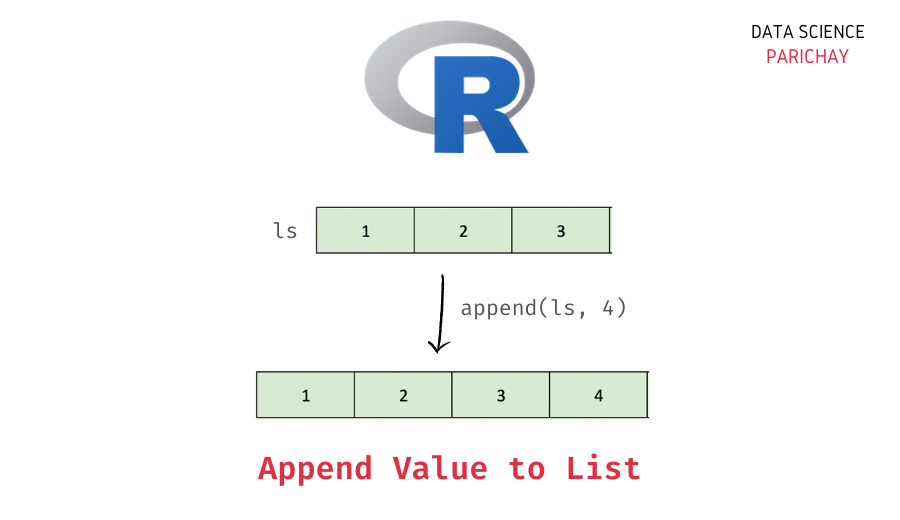# Append Element to a List in R

A list is an in-built data structure in R used to store one-dimensional data. A list, unlike a vector, can store values of different data types together. When working with lists, it can be handy to know how to quickly append value to them. In this tutorial, we will look at how to append an element to an existing list in R with the help of some examples.

## How to append an element to a list in R?

You can use the R `append()` function to append a value to a list. Pass the list and the value as arguments to the function. The following is the syntax.

```# append values to a list in R
append(x, values, after)```

It returns a list with the appended value. Let’s take a closer look at the arguments for this function.

• x – The list to which you want to append the value(s).
• values – The value (or values) you want to append.
• after – (Optional) The index after which to append the value in the list. It is equal to the length of the list by default. That is, by default, it appends the value(s) to the end of the list.

## Examples

Let’s look at some examples of using the above syntax to add a value to a list in R. First, we will create a list that we will be using throughout this tutorial.

```# create a list
ls <- list(1, 2, 3)
# display the list
print(ls)```

Output:

```[]
 1

[]
 2

[]
 3```

We now have a list of some integer values.

### Append value to a list in R

By default, the `append()` function adds the value to the end (that is, it appends the value). For example, let’s append the value 4 to the above list. For this, Pass the list and the value to append as arguments to the `append()` function.

```# append 4 to the list
ls <- append(ls, 4)
# display the list
print(ls)```

Output:

📚 Data Science Programs By Skill Level

Introductory

Intermediate ⭐⭐⭐

🔎 Find Data Science Programs 👨‍💻 111,889 already enrolled

Disclaimer: Data Science Parichay is reader supported. When you purchase a course through a link on this site, we may earn a small commission at no additional cost to you. Earned commissions help support this website and its team of writers.

```[]
 1

[]
 2

[]
 3

[]
 4```

You can see that the resulting list has the value 4 at the end.

### Append element at a given index in a list in R

The `append()` function also has an additional parameter, `after` using which you can specify the index after which you want to append the given element. For example, let’s append 5 to the above list (having values from 1 to 4) after index 2.

```# append value after specific index
ls <- append(ls, 5, 2)
# display the list
print(ls)```

Output:

```[]
 1

[]
 2

[]
 5

[]
 3

[]
 4```

You can see that the value 5 is added to the list in the 3rd index (that is after index 2).

In this tutorial, we looked at how the `append()` function can be used to append a value to a list. This function also allows you to append a value after a custom index in the list.

You might also be interested in –

•